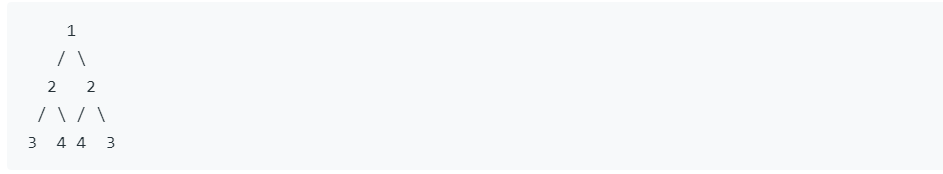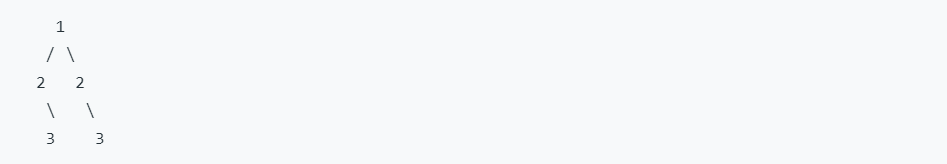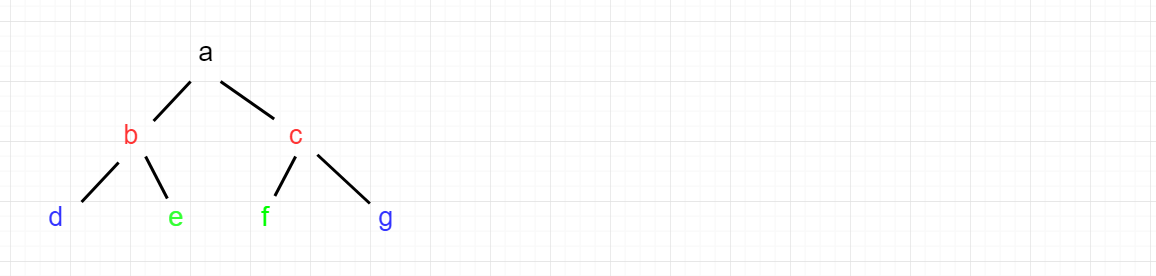• 请实现一个函数，用来判断一个二叉树是不是对称的。 注意 如果一个二叉树通次二叉树的镜像是相同的，则定义其实对称的。 题目： /* struct TreeNode { int val; struct TreeNode *left; struct TreeNode *...


–
–

题目描述
请实现一个函数，用来判断一个二叉树是不是对称的。

注意
如果一个二叉树通次二叉树的镜像是相同的，则定义其实对称的。

题目：
/*
struct TreeNode {
int val;
struct TreeNode *left;
struct TreeNode *right;
TreeNode(int x) :
val(x), left(NULL), right(NULL) {
}
};
*/
class Solution {
public:
bool isSymmetrical(TreeNode* pRoot)
{

}
};


判断一个二叉树是否是对称的，我们可以利用递归的方法来进行判断
我们可以从根部节点来进行判断
1.判断左右两孩子结点均不为空，则判断两值是否相等，相等，继续向下判断。不相等，直接返回 false
2.判断左右俩孩子均为空，返回 true
3.判断左右俩孩子只有一个孩子为空，另一个不为空，返回 false

通过代码：
/*
struct TreeNode {
int val;
struct TreeNode *left;
struct TreeNode *right;
TreeNode(int x) :
val(x), left(NULL), right(NULL) {
}
};
*/
class Solution {
public:
bool decision(TreeNode* left, TreeNode* right)
{
//左右俩孩子结点均不为空
if(left != NULL && right != NULL)
{
//判断左孩子和右孩子值是否相等
if(left->val != right->val)
return false;
return decision(left->left, right->right) && decision(left->right, right->left);
}
//左右孩子结点均为空
if(left == NULL && right == NULL)
return true;
//其中一个为空
if(left != NULL && right == NULL)
return false;
if(left == NULL && right != NULL)
return false;
}

bool isSymmetrical(TreeNode* pRoot)
{
//当二叉树为空树的时候
if(!pRoot)
return true;
//当二叉树不为空树的时候
return decision(pRoot->left, pRoot->right);
}

};



展开全文对称
• 给定一棵二叉树判断琪是否是自身的镜像（即：是否对称） 例如：下面这棵二叉树对称的 1↵ / ↵ 2 2↵ / / ↵3 4 4 3↵ 下面这棵二叉树对称。 1↵ / ↵ 2 2↵ ↵ 3 3 思路：核心递归函数check...
题目描述

给定一棵二叉树，判断琪是否是自身的镜像（即：是否对称）

例如：下面这棵二叉树是对称的

1↵   / ↵  2   2↵ /  / ↵3  4 4  3↵

下面这棵二叉树不对称。

1↵   / ↵  2   2↵      ↵   3    3

思路：核心递归函数check(Node* p1,Node* p2)    判断p1->left  p2->right    以及  p1->right p2->left

   bool isSymmetric(TreeNode* root) {
// write code here

return check(root,root);
}

bool check(TreeNode* left,TreeNode* right)
{
if(left==NULL && right==NULL)
return true;
if((left==NULL && right!=NULL) || (left!=NULL && right==NULL))
return false;
return left->val==right->val && check(left->left,right->right) && check(left->right,right->left);

}



展开全文• 判断根节点 a 的左节点 b 和右节点 c 想不想等，不相等则返回false，相等的话，递归判断 b 的左子树 d 和 c 的右子树 g 是否相等、b 的右子树e 和 c 的左子树 f 是否相等，如果相等，继续递归。
题目
给定一个二叉树，检查它是否是镜像对称的。
例如，二叉树 [1,2,2,3,4,4,3] 是对称的。但是下面这个 [1,2,2,null,3,null,3] 则不是镜像对称的:说明:
如果你可以运用递归和迭代两种方法解决这个问题，会很加分。
解法
解题思路：
先判断根节点 a 的左节点 b 和右节点 c 想不想等，不相等则返回false，相等的话，递归判断 b 的左子树 d 和 c 的右子树 g 是否相等、b 的右子树 e 和 c 的左子树 f 是否相等，如果相等，继续递归。代码：
/**
* Definition for a binary tree node.
* public class TreeNode {
*     int val;
*     TreeNode left;
*     TreeNode right;
*     TreeNode(int x) { val = x; }
* }
*/
class Solution {
public boolean isSymmetric(TreeNode root) {
return isMirror(root,root);
}

public boolean isMirror(TreeNode root1,TreeNode root2) {

if (root1 == null && root2 == null) return true;
if (root1 == null || root2 == null) return false;
if(root1.val != root2.val) return false;

//如果是第一个根节点，直接比较左右子树，避免重复比较
if(root1 == root2) return isMirror(root1.left,root1.right);

return isMirror(root1.left,root2.right)&&
isMirror(root1.right,root2.left);
}
}



展开全文• 二叉树最小深度 递归 class Solution { public int minDepth(TreeNode root) { if(root==null) return 0; return helper(root); } public int helper(TreeNode root){ if(r...
求二叉树最小深度  递归
class Solution {
public int minDepth(TreeNode root) {
if(root==null) return 0;
return helper(root);

}
public int helper(TreeNode root){
if(root==null) return Integer.MAX_VALUE;//因为是最小深度 不能返回0
if(root.left==null && root.right==null) return 1;
return Math.min(helper( root.right),helper(root.left))+1;
}
}

一行代码解决：
class Solution {
public int maxDepth(TreeNode root) {
return root == null ? 0 : Math.max(maxDepth(root.left), maxDepth(root.right)) + 1;
}
}

反转二叉树
3.29自己写的
class Solution {
public TreeNode invertTree(TreeNode root) {
if(root==null) return null;
TreeNode tmp=root.left;
root.left=root.right;
root.right=tmp;
invertTree(root.left);
invertTree(root.right);
return root;
}
}

别人写的
class Solution {
public TreeNode invertTree(TreeNode root) {
if(root==null) return null;
invertTree(root.left);
invertTree(root.right);
TreeNode temp=root.left;
root.left=root.right;
root.right=temp;
return root;

}
}

另一种写法：
class Solution {
public TreeNode invertTree(TreeNode root) {
if(root==null) return null;
TreeNode left=invertTree(root.left);
TreeNode right=invertTree(root.right);
root.left=right;
root.right=left;
return root;

}
}

判断二叉树对称
自己3.29 写的
/**
* Definition for a binary tree node.
* public class TreeNode {
*     int val;
*     TreeNode left;
*     TreeNode right;
*     TreeNode(int x) { val = x; }
* }
*/
class Solution {
public boolean isSymmetric(TreeNode root) {
if(root==null) return true;
return helper(root,root);
}
private boolean helper(TreeNode left,TreeNode right){
if(right==null && left==null) return true;
if(right==null || left==null) return false;
if(left.val!=right.val) return false;
return helper(left.left,right.right) && helper(left.right,right.left);
}
}

递归
要把几个情况都想出来 false 的 true 的
class Solution {
public boolean isSymmetric(TreeNode root) {
if(root==null) return true;
return helper(root,root);
}
public boolean helper(TreeNode root1,TreeNode root2){
if(root1==null && root2==null) return true;
if(root1==null || root2==null) return false;
if(root1.val!=root2.val) return false;
return helper(root1.left,root2.right) && helper(root1.right,root2.left);
}
}

时间复杂度：O(n)，因为我们遍历整个输入树一次，所以总的运行时间为 O(n)，其中 n是树中结点的总数。
空间复杂度：递归调用的次数受树的高度限制。在最糟糕情况下，树是线性的，其高度为 O(n)。因此，在最糟糕的情况下，由栈上的递归调用造成的空间复杂度为 O(n)。
迭代
队列中每两个连续的结点应该是相等的，而且它们的子树互为镜像。
最初，队列中包含的是 root 以及 root。
该算法的工作原理类似于 BFS，但存在一些关键差异。每次提取两个结点并比较它们的值。
然后，将两个结点的左右子结点按相反的顺序插入队列中。当队列为空时，或者我们检测到树不对称（即从队列中取出两个不相等的连续结点）时，该算法结束。
public boolean isSymmetric(TreeNode root) {
while (!q.isEmpty()) {
TreeNode t1 = q.poll();
TreeNode t2 = q.poll();
if (t1 == null && t2 == null) continue;
if (t1 == null || t2 == null) return false;
if (t1.val != t2.val) return false;
}
return true;
}


思考：先判断 ，后添加，成对成对添加
求二叉树节点个数
给出一个完全二叉树，求出该树的节点个数。
说明：
完全二叉树的定义如下：在完全二叉树中，除了最底层节点可能没填满外，其余每层节点数都达到最大值，并且最下面一层的节点都集中在该层最左边的若干位置。若最底层为第 h 层，则该层包含 1~ 2h 个节点。
示例:
输入:
1
/
2   3
/ \  /
4  5 6
普通二叉树递归求个数
class Solution {
public int countNodes(TreeNode root) {
if(root==null) return 0;
int left=countNodes(root.left);
int right=countNodes(root.right);
return 1+left+right;
}
}

以上方法容易理解但是没有利用完全二叉树的特点
巧方法（3.29再思考思考）
首先需要明确完全二叉树的定义：它是一棵空树或者它的叶子节点只出在最后两层，若最后一层不满则叶子节点只在最左侧。
再来回顾一下满二叉的节点个数怎么计算，如果满二叉树的层数为h，则总节点数为：2^h - 1.
那么我们来对root节点的左右子树进行高度统计，分别记为left和right,有以下两种结果：

left == right。这说明，左子树一定是满二叉树，因为节点已经填充到右子树了，左子树必定已经填满了。所以左子树的节点总数我们可以直接得到，是2^left - 1，加上当前这个root节点，则正好是2^left。再对右子树进行递归统计。
left != right。说明此时最后一层不满，但倒数第二层已经满了，可以直接得到右子树的节点个数。同理，右子树节点+root节点，总数为2^right。再对左子树进行递归查找。
关于如何计算二叉树的层数，可以利用下面的递归来算，当然对于完全二叉树，可以利用其特点，不用递归直接算，具体请参考最后的完整代码。

如何计算2^left，最快的方法是移位计算，因为运算符的优先级问题，记得加括号哦。
class Solution {
public int countNodes(TreeNode root) {
if(root==null) return 0;
int left=countLevel(root.left);
int right=countLevel(root.right);
if(left==right) {
return countNodes(root.right)+(1<<left);
}else
return countNodes(root.left)+(1<<right);
}
public int countLevel(TreeNode root){
int level=0;
while(root!=null){
level++;
root=root.left;
}
return level;
}
}
/*


判断平衡二叉树
给定一个二叉树，判断它是否是高度平衡的二叉树。
本题中，一棵高度平衡二叉树定义为：
一个二叉树每个节点 的左右两个子树的高度差的绝对值不超过1。
示例 1:
给定二叉树 [3,9,20,null,null,15,7]
3

/
9  20
/
15   7
返回 true 。
递归
从底至顶（提前阻断法）
对二叉树做深度优先遍历DFS，递归过程中：
终止条件：当DFS越过叶子节点时，返回高度0；
返回值：
从底至顶，返回以每个节点root为根节点的子树最大高度(左右子树中最大的高度值加1max(left,right) + 1)；
当我们发现有一例 左/右子树高度差 ＞ 1 的情况时，代表此树不是平衡树，返回-1；
当发现不是平衡树时，后面的高度计算都没有意义了，因此一路返回-1，避免后续多余计算。
最差情况是对树做一遍完整DFS，时间复杂度为 O(N)。
class Solution {
public boolean isBalanced(TreeNode root) {
return depth(root) != -1;
}

private int depth(TreeNode root) {
if (root == null) return 0;
int left = depth(root.left);
if(left == -1) return -1;
int right = depth(root.right);
if(right == -1) return -1;
return Math.abs(left - right) < 2 ? Math.max(left, right) + 1 : -1;
}
}


class Solution {
public boolean isBalanced(TreeNode root) {
return depth(root)!=-1;
}
public int depth(TreeNode root){
if(root==null) return 0;
int left=depth(root.left);
int right=depth(root.right);
if(left==-1) return -1;
if(right==-1) return -1;
if(Math.abs(left-right)<2)
return Math.max(left,right)+1;
else
return -1;
}
}
/*



展开全文• 主要介绍了PHP实现判断二叉树是否对称的方法,涉及php递归二叉树判断节点的相关操作技巧,需要的朋友可以参考下
• 请实现一个函数，用来判断一颗二叉树是不是对称的。注意，如果一个二叉树同此二叉树的镜像是同样的，定义其为对称的。 /* struct TreeNode { int val; struct TreeNode *left; struct TreeNode *right; ...
• 这篇文章主要介绍了PHP实现判断二叉树是否对称的方法,涉及php递归二叉树判断节点的相关操作技巧,对PHP感谢的朋友可以参考下本篇文章本文实例讲述了PHP实现判断二叉树是否对称的方法。分享给大家供大家参考，具体如下...
• 这篇文章主要介绍了PHP实现判断二叉树是否对称的方法,涉及php递归二叉树判断节点的相关操作技巧,需要的朋友可以参考下本文实例讲述了PHP实现判断二叉树是否对称的方法。分享给大家供大家参考，具体如下：问题请实现...
• 判断二叉树是否对称 //判断二叉树是否对称 /*给定一棵二叉树，判断琪是否是自身的镜像（即：是否对称）*/ struct TreeNode { int val; struct TreeNode *left; struct TreeNode *right; }; class Solution { ...算法 数据结构
• 判断二叉树是否对称 分析 节点的左右子树为空，则直接返回true 节点的左右子树有一个为空，则直接返回false 节点的左右子树均不为空，则判断节点的左右子节点的值是否相等 代码 public static boolean isSymmetric...
• 判断二叉树对称性 给定一个二叉树，检查它是否是镜像对称的。 例如，二叉树 [1,2,2,3,4,4,3] 是对称的。 1 / \ 2 2 / \ / \ 3 4 4 3 但是下面这个 [1,2,2,null,3,null,3] 则不是镜像对称的: 1 / \ 2 2 \ ...
• 给定一个二叉树，检查它是否是镜像对称的。 例如，二叉树[1,2,2,3,4,4,3] 是对称的。 1 / \ 2 2 / \ / \ 3 4 4 3 但是下面这个[1,2,2,null,3,null,3] 则不是镜像对称的: 1 / \ 2 2 \ \ 3 3 解题...指针 leetcode 数据结构
• 本文实例讲述了PHP实现判断二叉树是否对称的方法。分享给大家供大家参考，具体如下：问题请实现一个函数，用来判断一颗二叉树是不是对称的。注意，如果一个二叉树同此二叉树的镜像是同样的，定义其为对称的。题解...
• 思路：要判断一颗二叉树是否对称，要判断一下几点，可以用递归来实现： 判断一颗二叉树是不是对称的，等价于判断其左右子树是不是镜像对称判断对称像即判断对称的位置上的元素是不是相等 两个节点A和B对称...
• // 根节点(都不为空的情况下)成镜像的情况下，判断左右儿子是否成镜像 if(t1!=NULL&&t2!=NULL) return isSymmetric2(t1->left,t2->right)&&isSymmetric2(t1->right,t2->left); else return false; } }; ...数据结构...